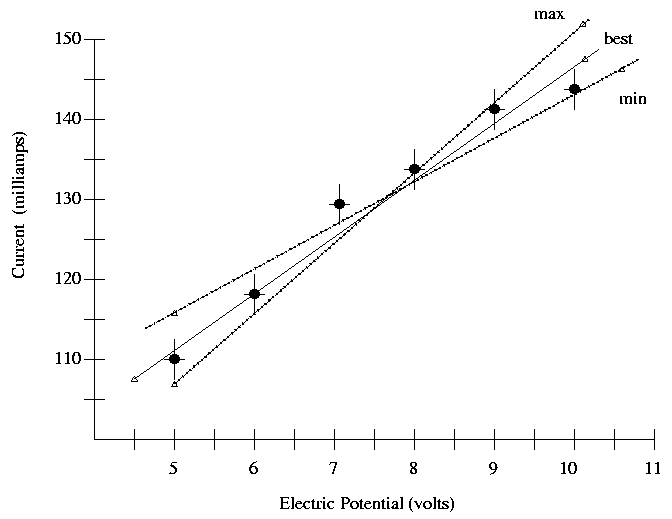Copyright © Michael Richmond. This work is licensed under a Creative Commons License.

Uncertainty in the slope on a graph

If one has more than a few points on a graph, one should calculate the uncertainty in the slope as follows. In the picture below, the data points are shown by small, filled, black circles; each datum has error bars to indicate the uncertainty in each measurement. It appears that current is measured to +/- 2.5 milliamps, and voltage to about +/- 0.1 volts. The hollow triangles represent points used to calculate slopes. Notice how I picked points near the ends of the lines to calculate the slopes!1. Draw the "best" line through all the points, taking into account the error bars. Measure the slope of this line.

2. Draw the "min" line -- the one with as small a slope as you think reasonable (taking into account error bars), while still doing a fair job of representing all the data. Measure the slope of this line.

3. Draw the "max" line -- the one with as large a slope as you think reasonable (taking into account error bars), while still doing a fair job of representing all the data. Measure the slope of this line.

4. Calculate the uncertainty in the slope as one-half of the difference between max and min slopes.

In the example above, I find

147 mA - 107 mA             mA
"best" slope   =   ------------------  =  7.27 ----
10 V  - 4.5 V               V

145 mA - 115 mA             mA
"min"  slope   =   ------------------  =  5.45 ----
10.5 V - 5.0 V               V

152 mA - 106 mA             mA
"max"  slope   =   ------------------  =  9.20 ----
10 V  - 5.0 V               V

mA
Uncertainty in slope is  0.5 * (9.20 - 5.45) =  1.875  ----
V

There are at most two significant digits in the slope, based on the uncertainty. So, I would say the graph shows

mA
slope  =   7.3  +/-  1.9   ----
VCopyright © Michael Richmond. This work is licensed under a Creative Commons License.